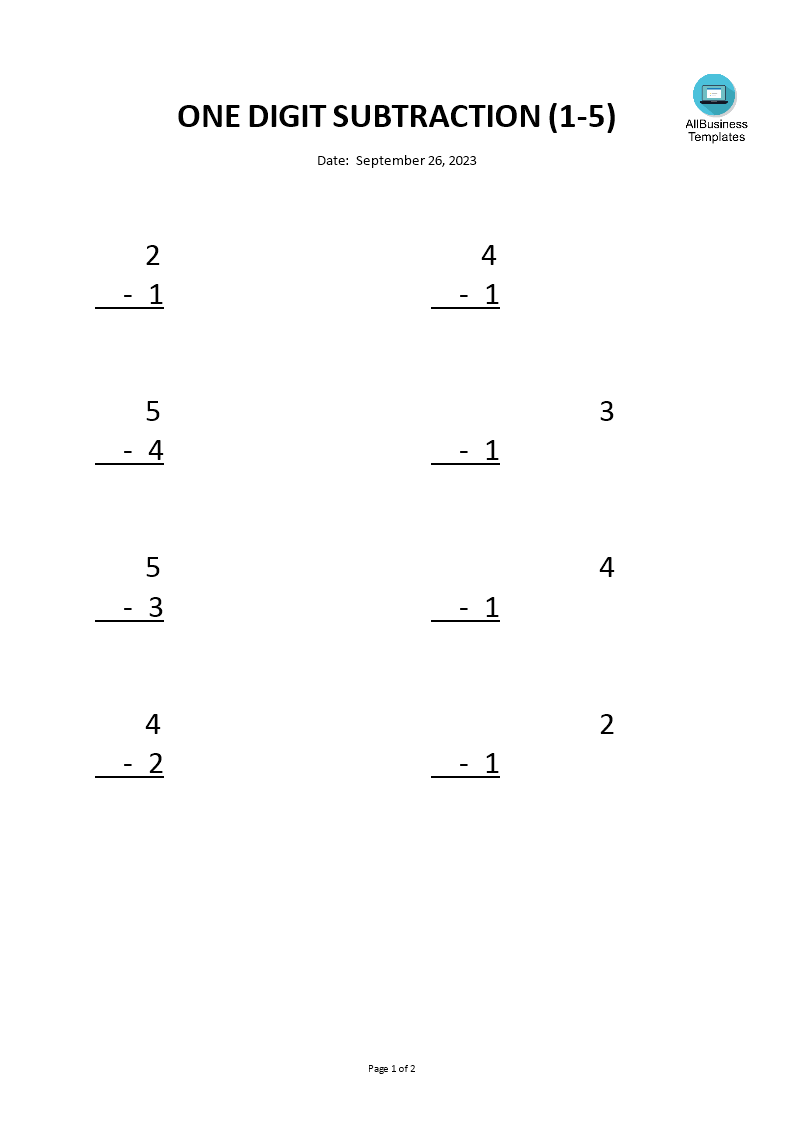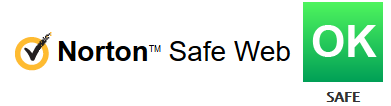# Subtraction 1 to 5 worksheets

Save, fill-In The Blanks, Print, Done!Click on image to zoom / Click button below to see more images

Microsoft Word (.docx)

Or select the format you want and we convert it for you for free:

• This Document Has Been Certified by a Professional
• 100% customizable
• Language: English

ABT template rating: 7

Malware- and virusfree. Scanned by:What is an example of subtraction for kids? How to make a simple subtraction worksheet? Download this sample worksheet template and you can easily create a subtraction for kids. The worksheet can be customized to meet the specific needs of your classroom.

A "One Digit 1 to 5 Subtraction Worksheet" is a specific type of subtraction practice sheet designed for young students who are just beginning to learn basic subtraction concepts. It focuses on subtracting one-digit numbers ranging from 1 to 5. These worksheets are typically used in early elementary education, often for kindergarten or first-grade students.

Here's what you might find on a One-Digit 1 to 5 Subtraction Worksheet:

1. Simple Subtraction Problems: The worksheet will contain a series of subtraction problems where the numbers involved are all one-digit numbers (e.g., 1, 2, 3, 4, 5).
2. Single-Digit Minuends and Subtrahends: The minuend (the number from which you subtract) and the subtrahend (the number being subtracted) will both be single-digit numbers.
3. Visual Aids: Depending on the age and skill level of the students, these worksheets might include visual aids such as pictures, dots, or objects to help students understand and count the numbers they are subtracting.
4. Space for Writing Answers: There will be space provided for students to write their answers to each subtraction problem.
5. Gradual Increase in Complexity: The difficulty of the problems may gradually increase as students progress through the worksheet. For example, it might start with simple problems like 5 - 2 and progress to slightly more challenging ones like 4 - 3.

The purpose of these worksheets is to introduce young learners to the concept of subtraction in a simple and accessible way. By focusing on small numbers and limited subtraction facts, students can build a foundational understanding of subtraction before moving on to more complex subtraction problems involving larger numbers. Practicing subtraction at a very young age will help kids to become more excellent in mathematics. Start practicing in kindergarten or at home. Make sure it's fun. This Subtraction Worksheet for kids is a good example of how to test them.

This 5-1 practice solving inequalities by addition and subtraction worksheet answers worksheet is intuitive, ready-to-use, and structured in a smart way. Try it now and let this template inspire you. We certainly encourage you to use this One-Digit 1 to 5 Subtraction Worksheet for your own good and are confident it will fit your needs.

Our site is updated every day with new Education Templates. Download this 1 to 5 Subtraction Worksheet now!

DISCLAIMER
Nothing on this site shall be considered legal advice and no attorney-client relationship is established.